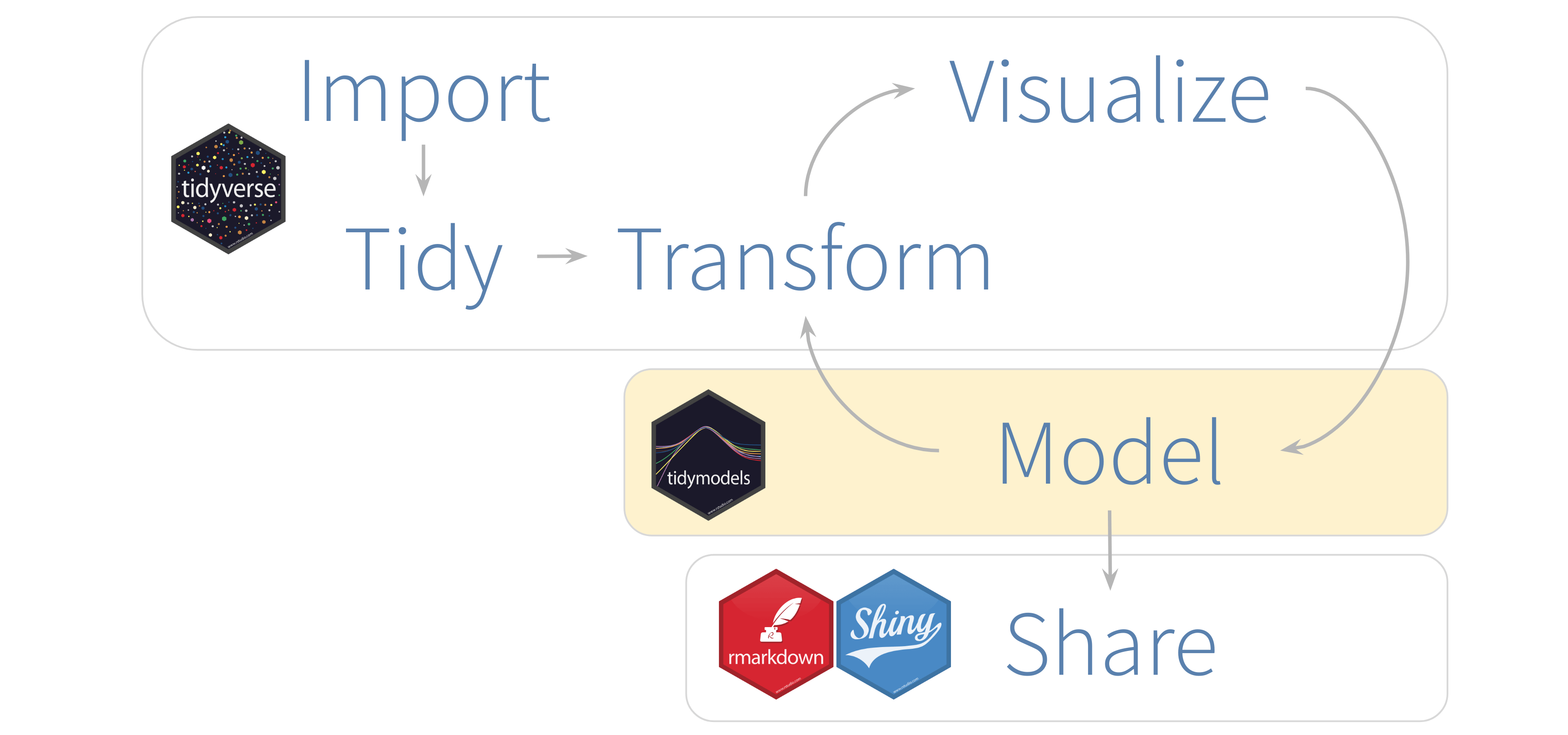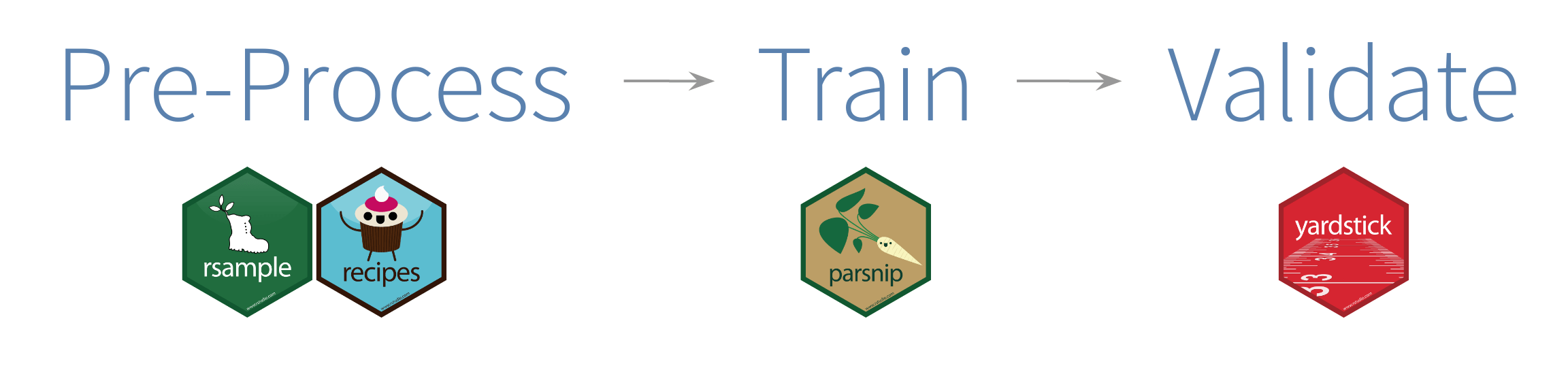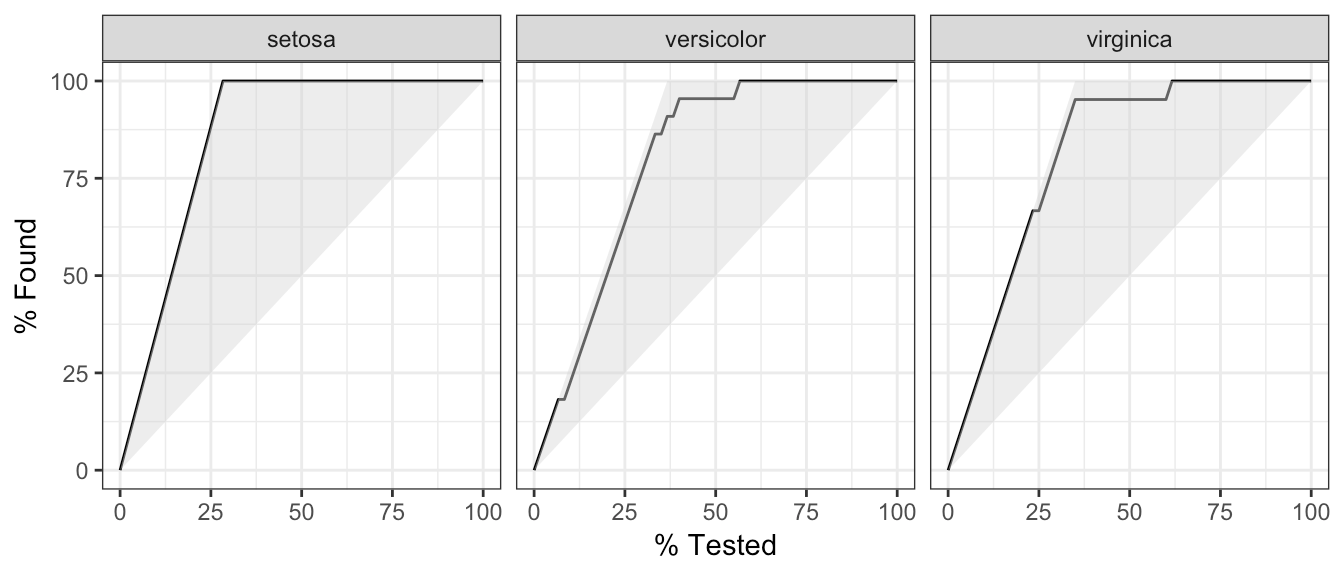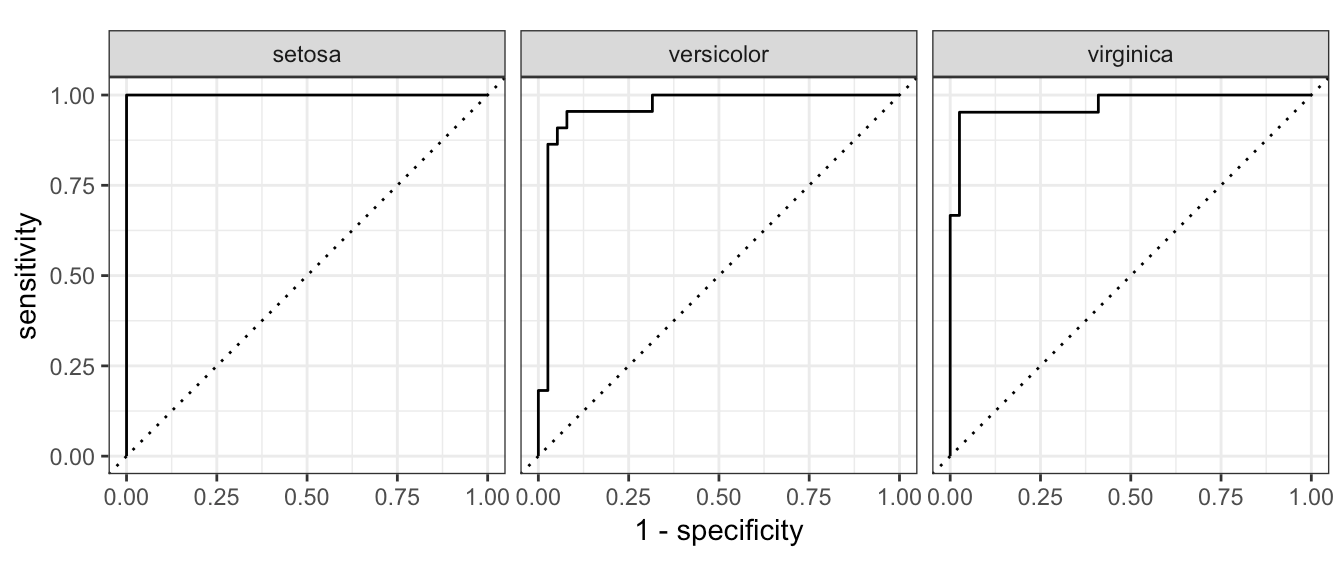Want to share your content on R-bloggers? click here if you have a blog, or here if you don't.Recently, I had the opportunity to showcase `tidymodels` in workshops and talks. Because of my vantage point as a user, I figured it would be valuable to share what I have learned so far. Let’s begin by framing where `tidymodels` fits in our analysis projects.

The diagram above is based on the R for Data Science book, by Wickham and Grolemund. The version in this article illustrates what step each package covers. Even though it is a single step, developing models can benefit from having a `tidyverse`-friendly interface. That is where `tidymodels` comes in.

It is important to clarify that the group of packages that make up `tidymodels` do not implement statistical models themselves. Instead, they focus on making all the tasks around fitting the model much easier. Those tasks are data pre-processing and results validation.

In a way, the Model step itself has sub-steps. For these sub-steps, `tidymodels` provides one or several packages. This article will showcase functions from four `tidymodels` packages:

• `rsample` – Different types of re-samples
• `recipes` – Transformations for model data pre-processing
• `parnip` – A common interface for model creation
• `yardstick` – Measure model performance

The following diagram illustrates each modeling step, and lines up the `tidymodels` packages that we will use in this article:In a given analysis, a `tidyverse` package may or may not be used. Not all projects need to work with time variables, so there is no need to use functions from the `hms` package. The same idea applies to `tidymodels`. Depending on what type of modeling is going to be done, only functions from some its packages will be used.

## An Example

We will use the `iris` data set for an example. Its data is already imported, and sufficiently tidy to move directly to modeling.

### Load only the `tidymodels` library

This may be the first article I have written where only one package is called via `library()`. Apart from loading its core modeling packages, `tidymodels` also conveniently loads some `tidyverse` packages, including `dplyr` and `ggplot2`. Throughout this exercise, we will use some functions out of those packages, but we don’t have to explicitly load them into our R session.

`library(tidymodels)`

### Pre-Process

This step focuses on making data suitable for modeling by using data transformations. All transformations can be accomplished with `dplyr`, or other `tidyverse` packages Consider using `tidymodels` packages when model development is more heavy and complex.

#### Data Sampling

The `initial_split()` function is specially built to separate the data set into a training and testing set. By default, it holds 3/4 of the data for training and the rest for testing. That can be changed by passing the `prop` argument. This function generates an `rplit` object, not a data frame. The printed output shows the row count for testing, training, and total.

```iris_split <- initial_split(iris, prop = 0.6)
iris_split```

`## <90/60/150>`

To access the observations reserved for training, use the `training()` function. Similarly, use `testing()` to access the testing data.

```iris_split %>%
training() %>%
glimpse()```

```## Observations: 90
## Variables: 5
## \$ Sepal.Length <dbl> 5.1, 4.9, 4.7, 4.6, 5.0, 5.4, 4.6, 5.0, 4.9, 5.4, 4…
## \$ Sepal.Width  <dbl> 3.5, 3.0, 3.2, 3.1, 3.6, 3.9, 3.4, 3.4, 3.1, 3.7, 3…
## \$ Petal.Length <dbl> 1.4, 1.4, 1.3, 1.5, 1.4, 1.7, 1.4, 1.5, 1.5, 1.5, 1…
## \$ Petal.Width  <dbl> 0.2, 0.2, 0.2, 0.2, 0.2, 0.4, 0.3, 0.2, 0.1, 0.2, 0…
## \$ Species      <fct> setosa, setosa, setosa, setosa, setosa, setosa, set…```

These sampling functions are courtesy of the `rsample` package, which is part of `tidymodels`.

#### Pre-process interface

In `tidymodels`, the `recipes` package provides an interface that specializes in data pre-processing. Within the package, the functions that start, or execute, the data transformations are named after cooking actions. That makes the interface more user-friendly. For example:

• `recipe()` – Starts a new set of transformations to be applied, similar to the `ggplot()` command. Its main argument is the model’s formula.

• `prep()` – Executes the transformations on top of the data that is supplied (typically, the training data).

Each data transformation is a step. Functions correspond to specific types of steps, each of which has a prefix of `step_`. There are several `step_` functions; in this example, we will use three of them:

• `step_corr()` – Removes variables that have large absolute correlations with other variables

• `step_center()` – Normalizes numeric data to have a mean of zero

• `step_scale()` – Normalizes numeric data to have a standard deviation of one

Another nice feature is that the step can be applied to a specific variable, groups of variables, or all variables. The `all_outocomes()` and `all_predictors()` functions provide a very convenient way to specify groups of variables. For example, if we want the `step_corr()` to only analyze the predictor variables, we use `step_corr(all_predictors())`. This capability saves us from having to enumerate each variable.

In the following example, we will put together the `recipe()`, `prep()`, and step functions to create a `recipe` object. The `training()` function is used to extract that data set from the previously created split sample data set.

```iris_recipe <- training(iris_split) %>%
recipe(Species ~.) %>%
step_corr(all_predictors()) %>%
step_center(all_predictors(), -all_outcomes()) %>%
step_scale(all_predictors(), -all_outcomes()) %>%
prep()```

If we call the `iris_recipe` object, it will print details about the recipe. The Operations section describes what was done to the data. One of the operations entries in the example explains that the correlation step removed the `Petal.Length` variable.

`iris_recipe`

```## Data Recipe
##
## Inputs:
##
##       role #variables
##    outcome          1
##  predictor          4
##
## Training data contained 90 data points and no missing data.
##
## Operations:
##
## Correlation filter removed Petal.Length [trained]
## Centering for Sepal.Length, Sepal.Width, Petal.Width [trained]
## Scaling for Sepal.Length, Sepal.Width, Petal.Width [trained]```

### Execute the pre-processing

The testing data can now be transformed using the exact same steps, weights, and categorization used to pre-process the training data. To do this, another function with a cooking term is used: `bake()`. Notice that the `testing()` function is used in order to extract the appropriate data set.

```iris_testing <- iris_recipe %>%
bake(testing(iris_split))

glimpse(iris_testing)```

```## Observations: 60
## Variables: 4
## \$ Sepal.Length <dbl> -1.597601746, -1.138960096, 0.007644027, -0.7949788…
## \$ Sepal.Width  <dbl> -0.41010139, 0.71517681, 2.06551064, 1.61539936, 0.…
## \$ Petal.Width  <dbl> -1.2085003, -1.2085003, -1.2085003, -1.0796318, -1.…
## \$ Species      <fct> setosa, setosa, setosa, setosa, setosa, setosa, set…```

Performing the same operation over the training data is redundant, because that data has already been prepped. To load the prepared training data into a variable, we use `juice()`. It will extract the data from the `iris_recipe` object.

```iris_training <- juice(iris_recipe)

glimpse(iris_training)```

```## Observations: 90
## Variables: 4
## \$ Sepal.Length <dbl> -0.7949789, -1.0242997, -1.2536205, -1.3682809, -0.…
## \$ Sepal.Width  <dbl> 0.94023245, -0.18504575, 0.26506553, 0.04000989, 1.…
## \$ Petal.Width  <dbl> -1.2085003, -1.2085003, -1.2085003, -1.2085003, -1.…
## \$ Species      <fct> setosa, setosa, setosa, setosa, setosa, setosa, set…```

### Model Training

In R, there are multiple packages that fit the same type of model. It is common for each package to provide a unique interface. In other words, things such as an argument for the same model attribute is defined differently for each package. For example, the `ranger` and `randomForest` packages fit Random Forest models. In the `ranger()` function, to define the number of trees we use `num.trees`. In `randomForest`, that argument is named `ntree`. It is not easy to switch between packages to run the same model.

Instead of replacing the modeling package, `tidymodels` replaces the interface. Better said, `tidymodels` provides a single set of functions and arguments to define a model. It then fits the model against the requested modeling package.

In the example below, the `rand_forest()` function is used to initialize a Random Forest model. To define the number of trees, the `trees` argument is used. To use the `ranger` version of Random Forest, the `set_engine()` function is used. Finally, to execute the model, the `fit()` function is used. The expected arguments are the formula and data. Notice that the model runs on top of the juiced trained data.

```iris_ranger <- rand_forest(trees = 100, mode = "classification") %>%
set_engine("ranger") %>%
fit(Species ~ ., data = iris_training)```

The payoff is that if we now want to run the same model against `randomForest`, we simply change the value in `set_engine()` to “randomForest”.

```iris_rf <-  rand_forest(trees = 100, mode = "classification") %>%
set_engine("randomForest") %>%
fit(Species ~ ., data = iris_training)```

It is also worth mentioning that the model is not defined in a single, large function with a lot of arguments. The model definition is separated into smaller functions such as `fit()` and `set_engine()`. This allows for a more flexible – and easier to learn – interface.

### Predictions

Instead of a vector, the `predict()` function ran against a `parsnip` model returns a `tibble`. By default, the prediction variable is called `.pred_class`. In the example, notice that the baked testing data is used.

`predict(iris_ranger, iris_testing)`

```## # A tibble: 60 x 1
##    .pred_class
##    <fct>
##  1 setosa
##  2 setosa
##  3 setosa
##  4 setosa
##  5 setosa
##  6 setosa
##  7 setosa
##  8 setosa
##  9 setosa
## 10 setosa
## # … with 50 more rows```

It is very easy to add the predictions to the baked testing data by using `dplyr`’s `bind_cols()` function.

```iris_ranger %>%
predict(iris_testing) %>%
bind_cols(iris_testing) %>%
glimpse()```

```## Observations: 60
## Variables: 5
## \$ .pred_class  <fct> setosa, setosa, setosa, setosa, setosa, setosa, set…
## \$ Sepal.Length <dbl> -1.597601746, -1.138960096, 0.007644027, -0.7949788…
## \$ Sepal.Width  <dbl> -0.41010139, 0.71517681, 2.06551064, 1.61539936, 0.…
## \$ Petal.Width  <dbl> -1.2085003, -1.2085003, -1.2085003, -1.0796318, -1.…
## \$ Species      <fct> setosa, setosa, setosa, setosa, setosa, setosa, set…```

### Model Validation

Use the `metrics()` function to measure the performance of the model. It will automatically choose metrics appropriate for a given type of model. The function expects a `tibble` that contains the actual results (`truth`) and what the model predicted (`estimate`).

```iris_ranger %>%
predict(iris_testing) %>%
bind_cols(iris_testing) %>%
metrics(truth = Species, estimate = .pred_class)```

```## # A tibble: 2 x 3
##   .metric  .estimator .estimate
##   <chr>    <chr>          <dbl>
## 1 accuracy multiclass     0.917
## 2 kap      multiclass     0.874```

Because of the consistency of the new interface, measuring the same metrics against the `randomForest` model is as easy as replacing the model variable at the top of the code.

```iris_rf %>%
predict(iris_testing) %>%
bind_cols(iris_testing) %>%
metrics(truth = Species, estimate = .pred_class)```

```## # A tibble: 2 x 3
##   .metric  .estimator .estimate
##   <chr>    <chr>          <dbl>
## 1 accuracy multiclass     0.883
## 2 kap      multiclass     0.824```

#### Per classifier metrics

It is easy to obtain the probability for each possible predicted value by setting the `type` argument to `prob`. That will return a `tibble` with as many variables as there are possible predicted values. Their name will default to the original value name, prefixed with `.pred_`.

```iris_ranger %>%
predict(iris_testing, type = "prob") %>%
glimpse()```

```## Observations: 60
## Variables: 3
## \$ .pred_setosa     <dbl> 0.677480159, 0.978293651, 0.783250000, 0.983972…
## \$ .pred_versicolor <dbl> 0.295507937, 0.011706349, 0.150833333, 0.001111…
## \$ .pred_virginica  <dbl> 0.02701190, 0.01000000, 0.06591667, 0.01491667,…```

Again, use `bind_cols()` to append the predictions to the baked testing data set.

```iris_probs <- iris_ranger %>%
predict(iris_testing, type = "prob") %>%
bind_cols(iris_testing)

glimpse(iris_probs)```

```## Observations: 60
## Variables: 7
## \$ .pred_setosa     <dbl> 0.677480159, 0.978293651, 0.783250000, 0.983972…
## \$ .pred_versicolor <dbl> 0.295507937, 0.011706349, 0.150833333, 0.001111…
## \$ .pred_virginica  <dbl> 0.02701190, 0.01000000, 0.06591667, 0.01491667,…
## \$ Sepal.Length     <dbl> -1.597601746, -1.138960096, 0.007644027, -0.794…
## \$ Sepal.Width      <dbl> -0.41010139, 0.71517681, 2.06551064, 1.61539936…
## \$ Petal.Width      <dbl> -1.2085003, -1.2085003, -1.2085003, -1.0796318,…
## \$ Species          <fct> setosa, setosa, setosa, setosa, setosa, setosa,…```

Now that everything is in one `tibble`, it is easy to calculate curve methods. In this case we are using `gain_curve()`.

```iris_probs%>%
gain_curve(Species, .pred_setosa:.pred_virginica) %>%
glimpse()```

```## Observations: 141
## Variables: 5
## \$ .level          <chr> "setosa", "setosa", "setosa", "setosa", "setosa"…
## \$ .n              <dbl> 0, 1, 3, 4, 5, 7, 8, 9, 10, 12, 13, 14, 15, 16, …
## \$ .n_events       <dbl> 0, 1, 3, 4, 5, 7, 8, 9, 10, 12, 13, 14, 15, 16, …
## \$ .percent_tested <dbl> 0.000000, 1.666667, 5.000000, 6.666667, 8.333333…
## \$ .percent_found  <dbl> 0.000000, 5.882353, 17.647059, 23.529412, 29.411…```

The curve methods include an `autoplot()` function that easily creates a `ggplot2` visualization.

```iris_probs%>%
gain_curve(Species, .pred_setosa:.pred_virginica) %>%
autoplot()```This is an example of a `roc_curve()`. Again, because of the consistency of the interface, only the function name needs to be modified; even the argument values remain the same.

```iris_probs%>%
roc_curve(Species, .pred_setosa:.pred_virginica) %>%
autoplot()```To measured the combined single predicted value and the probability of each possible value, combine the two prediction modes (with and without `prob` type). In this example, using `dplyr`’s `select()` makes the resulting `tibble` easier to read.

```predict(iris_ranger, iris_testing, type = "prob") %>%
bind_cols(predict(iris_ranger, iris_testing)) %>%
bind_cols(select(iris_testing, Species)) %>%
glimpse()```

```## Observations: 60
## Variables: 5
## \$ .pred_setosa     <dbl> 0.677480159, 0.978293651, 0.783250000, 0.983972…
## \$ .pred_versicolor <dbl> 0.295507937, 0.011706349, 0.150833333, 0.001111…
## \$ .pred_virginica  <dbl> 0.02701190, 0.01000000, 0.06591667, 0.01491667,…
## \$ .pred_class      <fct> setosa, setosa, setosa, setosa, setosa, setosa,…
## \$ Species          <fct> setosa, setosa, setosa, setosa, setosa, setosa,…```

Pipe the resulting table into `metrics()`. In this case, specify `.pred_class` as the `estimate`.

```predict(iris_ranger, iris_testing, type = "prob") %>%
bind_cols(predict(iris_ranger, iris_testing)) %>%
bind_cols(select(iris_testing, Species)) %>%
metrics(Species, .pred_setosa:.pred_virginica, estimate = .pred_class)```

```## # A tibble: 4 x 3
##   .metric     .estimator .estimate
##   <chr>       <chr>          <dbl>
## 1 accuracy    multiclass     0.917
## 2 kap         multiclass     0.874
## 3 mn_log_loss multiclass     0.274
## 4 roc_auc     hand_till      0.980```

## Closing remarks

This end-to-end example is intended to be a gentle introduction to `tidymodels`. The number of functions, and options of such functions, were kept at a minimum for the purposes of this demonstration, but there is much more that can be done with this wonderful group of packages. Hopefully, this article will help you get started, and maybe even encourage you to expand your knowledge further.

## Thank you!

I would like to thank Max Kuhn and Davis Vaughan, the primary developers of `tidymodels`. They have been very gracious in providing instruction, feedback, and guidance throughout my journey of learning `tidymodels`.